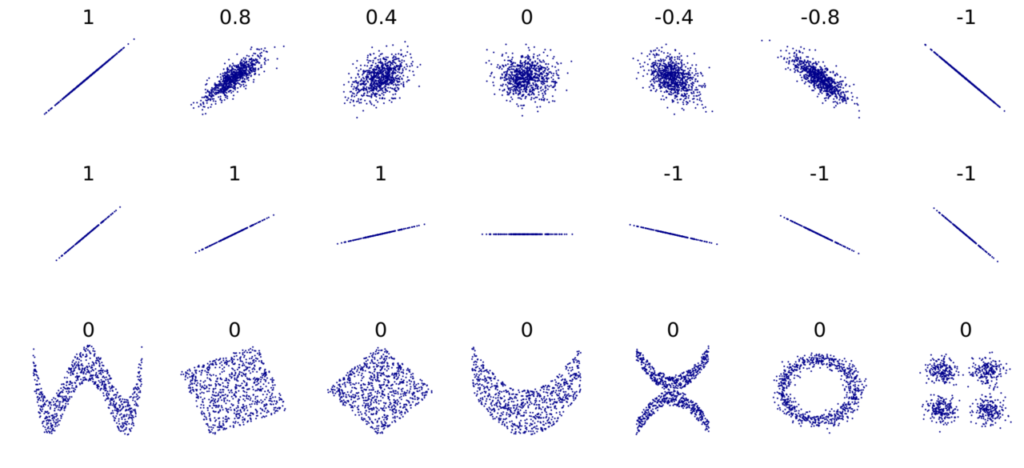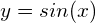# Does Causality imply Correlation?

I have seen enough threads saying that Correlation does NOT imply Causality. Yes, that is true, but how about the other way around? Have you thought about whether causation should be followed by correlation? This is the topic we are going to discuss in this blog post today.

Firstly, let’s make the definitions clear.

Causality is the phenomenon of, according to Wiki,

one process or state, a cause, contributes to the production of another process or state, an effect, where the cause is partly responsible for the effect, and the effect is partly dependent on the cause.

Correlation, also from Wiki,

is any statistical relationship, whether causal or not, between two random variables or bivariate data. In the broadest sense correlation is any statistical association, though it commonly refers to the degree to which a pair of variables are linearly related.

An important point here is: we normally perceive linear correlation as correlation, which is not so true as correlation denotes any relationship, including both linear and non-linear ones. The most popular correlation coefficient is Pearson’s, which only measures linear relationships. In the same wiki page, there is a figure depicting this point:Pearson correlation is affected by noise and the direction of linear relationships (top row), but not the slope (middle row). On the other hand, non-linear relationships give zero Pearson correlation coefficient (bottom row).

The fact is a lot of software programs implicitly use Pearson correlation when we ask them to calculate the correlation, which misleads us to think that the output (of Pearson correlation) represents the true (both linear and non-linear) correlation. Look at the Python code below:

```import numpy as np
x = np.linspace(0, 10000, 1000)
y = np.sin(x)
np.corrcoef(x, y)[0, 1]```
`-0.00035693847275798044`

The corrcoef function from Numpy, which says nothing about using Pearson correlation in its name, does represent the Pearson correlation, which in turn is only accounted for linear relationships. Hence, even though the variable y in the above scripts totally depends on x (), the outputted correlation is approximately zero.

Note that in its docs, they do give a clear statement about using Pearson correlation to compute the result of corrcoef, but since the statement is in the docs but not the name of the function, there are many reasons for us to overlook or forget that fact.

To give the first conclusion about what we have been talking about,

If by correlation, you mean linear correlation, then causality does NOT imply that correlation.

The counter-examples are the figure and the relationshipabove.

From this point on, to not be confused, when I use the word correlation, what I mean is the true correlation, the one includes both linear and non-linear relationships.

Return back to our main topic: Does Causality imply Correlation?

The answer is NO. Below is a counter-example:

Suppose you have a house with an air-conditioner inside. Normally, we know that the outside temperature affects the inside temperature of the house, this is undoubtedly causality.

Outside temperatureInside temperature

However, as you turn on the air-conditioner and set it to 20C, it keeps the inside temperature as a constant of 20C irrespective of the outside temperature. Now, when the outside temperature goes up (above 20C), the air-conditioner works harder, the inside temperature stays still. When the inside temperature goes down (below 20C), the air-conditioner also works harder, the inside temperature is still 20C.

This indicates that the inside temperature does not have any correlation with the outside temperature at all, it keeps being a constant (20C) when the outside temperature increases and decreases, even though there is a causality relationship between them.

The reason for this is the existence of a new variable, the air-conditioner, which also has a causality relationship with the inside temperature,

Air-conditionerInside temperature

and this force is completely opposite to the force from the outside temperature, thus these 2 forces cancel out each other.

To generalize, one object can be caused by many other objects (many causality relationships), each of the causal objects can have some different effects on the affected object. In some cases, when some of the causal objects make opposite effects on the affected object, they can cancel out each other, which results in zero correlation with the affected object.

We conclude it in a sentence:

Causality does NOT ensure correlation.

References: